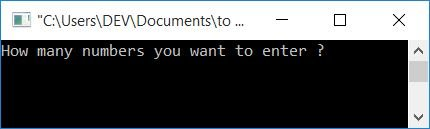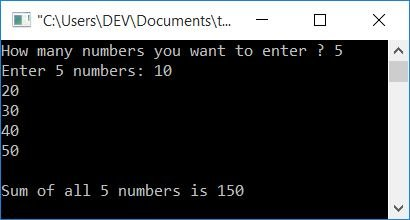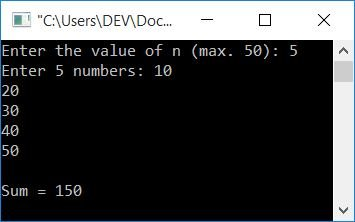# C++ Program to Add n Numbers

In this article, you will learn and get code for adding n numbers (or finding the sum of n numbers) given by the user at run-time using a C++ program. For example, if the user enters the value of n as 5, The program then asks you to enter any five numbers, so that it can add all the five entered numbers and print its summation result as output.

Here is the list of approaches that are used to do the task of adding n numbers:

To add n numbers in C++ programming, you have to ask the user to enter the value of n (i.e., how many numbers he/she wants to enter), then ask to enter n numbers to perform the addition of all the given numbers, and finally display the result on the screen as shown here in the following program.

## Using a for loop, calculate the sum of n numbers

This program is created using a for loop to do the job. The question is: write a program in C++ to find and print the sum of n numbers using a for loop. And here is the answer to this question:

```#include<iostream>
using namespace std;
int main()
{
int i, n, num, sum=0;
cout<<"How many numbers you want to enter ? ";
cin>>n;
cout<<"Enter "<<n<<" numbers: ";
for(i=0; i<n; i++)
{
cin>>num;
sum = sum+num;
}
cout<<"\nSum of all "<<n<<" numbers is "<<sum;
cout<<endl;
return 0;
}```

This program was built and runs under the Code::Blocks IDE. Here is its sample run:Now supply or enter the value of n (that is, how many numbers you want to enter and add them up), say 5. And then enter any five numbers. Press the ENTER key to see the following output, which is the sum of all the 5 numbers entered by the user at run-time:The program is very simple. That is, I received the value of n first and then created a for loop that runs n times to get all the numbers and add them up one by one. Therefore, if the user enters the value of n as 5, then the dry run of the above program goes like this:

• Inside the for loop, initially 0 gets initialized to i, and checks the condition, which is whether the value of i is less than n's value or not.
• Condition evaluates to be true, therefore program flow goes inside the loop and receives a number inside a variable, say num.
• And sum+num gets initialized to sum every time after receiving the number.
• Because the initial value of sum is 0, suppose the user enters 10 as the first number in the for loop. As a result, 0+10 or 10 is initialized to sum. Now sum=10.
• Program flow goes to the update part and increments the value of i and again checks the condition.
• The condition again evaluates to being true for the second time, and the program flow again goes inside the loop and executes the two statements. That is, it receives a number, say 20, and initializes sum + 20 or 10 + 20 as the new value of sum. As a result, sum = 30.
• In a similar way, process the operation.
• The loop's execution terminates, when its condition evaluated to be false.
• After we leave the loop, we'll have a variable called "sum" that holds the sum of all n numbers that the user has entered.
• Therefore, simply prints its value as output.

### What if the number has a decimal point?

To handle real numbers (numbers with a decimal part), use the float data type instead of int. Therefore, just replace the following statement as given in the above program:

`int i, n, num, sum=0;`

with the statement given below:

`float i, n, num, sum=0;`

Everything else will remain the same.

## Using a while loop, compute the sum of n numbers

The question is: write a program in C++ that finds and prints the sum of n given numbers using a while loop. The answer to this question is given below:

```#include<iostream>
using namespace std;
int main()
{
int n, i=0, num, sum=0;
cout<<"Enter the value of n: ";
cin>>n;
cout<<"Enter "<<n<<" numbers: ";
while(i<n)
{
cin>>num;
sum = sum+num;
i++;
}
cout<<"\nSum = "<<sum;
cout<<endl;
return 0;
}```

The output of the preceding program is as follows:Unlike for a loop, in a while loop we have to initialize the loop variable before the start of the loop. As it only contains the condition statement, we also have to place the update part inside the loop's body. Therefore, the value of i gets initialized before the loop, and its value gets updated using the last statement of its body.

## Using an Array, add n numbers

This program uses an array to do the same job as the previous program.

```#include<iostream>
using namespace std;
int main()
{
int n, i, arr, sum=0;
cout<<"Enter the value of n (max. 50): ";
cin>>n;
cout<<"Enter "<<n<<" numbers: ";
for(i=0; i<n; i++)
{
cin>>arr[i];
sum = sum+arr[i];
}
cout<<"\nSum = "<<sum;
cout<<endl;
return 0;
}```

Here is its sample run with the same input as the previous output:All the numbers get stored inside the array in a way that

• The first number is saved in arr.
• The second number is saved in arr.
• The third number is saved in arr.
• and so on.

## Using Function, Add n Numbers

This is the last program on adding n numbers. This program is created using a user-defined function named findSum() to do the same job. This function receives an array and its size as its two arguments, or parameters. The array is the list of numbers, and size is the value of n. Further, it finds the sum of all numbers in the list and returns it. That is, its return value gets initialized to sum inside the main() function:

```#include<iostream>
using namespace std;
int findSum(int [], int);
int main()
{
int n, i, arr, sum;
cout<<"Enter the value of n (max. 50): ";
cin>>n;
cout<<"Enter "<<n<<" numbers: ";
for(i=0; i<n; i++)
cin>>arr[i];
sum = findSum(arr, n);
cout<<"\nSum = "<<sum;
cout<<endl;
return 0;
}
int findSum(int arr[], int n)
{
int i, sum=0;
for(i=0; i<n; i++)
sum = sum+arr[i];
return sum;
}```

This will produce the same output as the previous one.

#### The same program in different languages

C++ Quiz

« Previous Program Next Program »